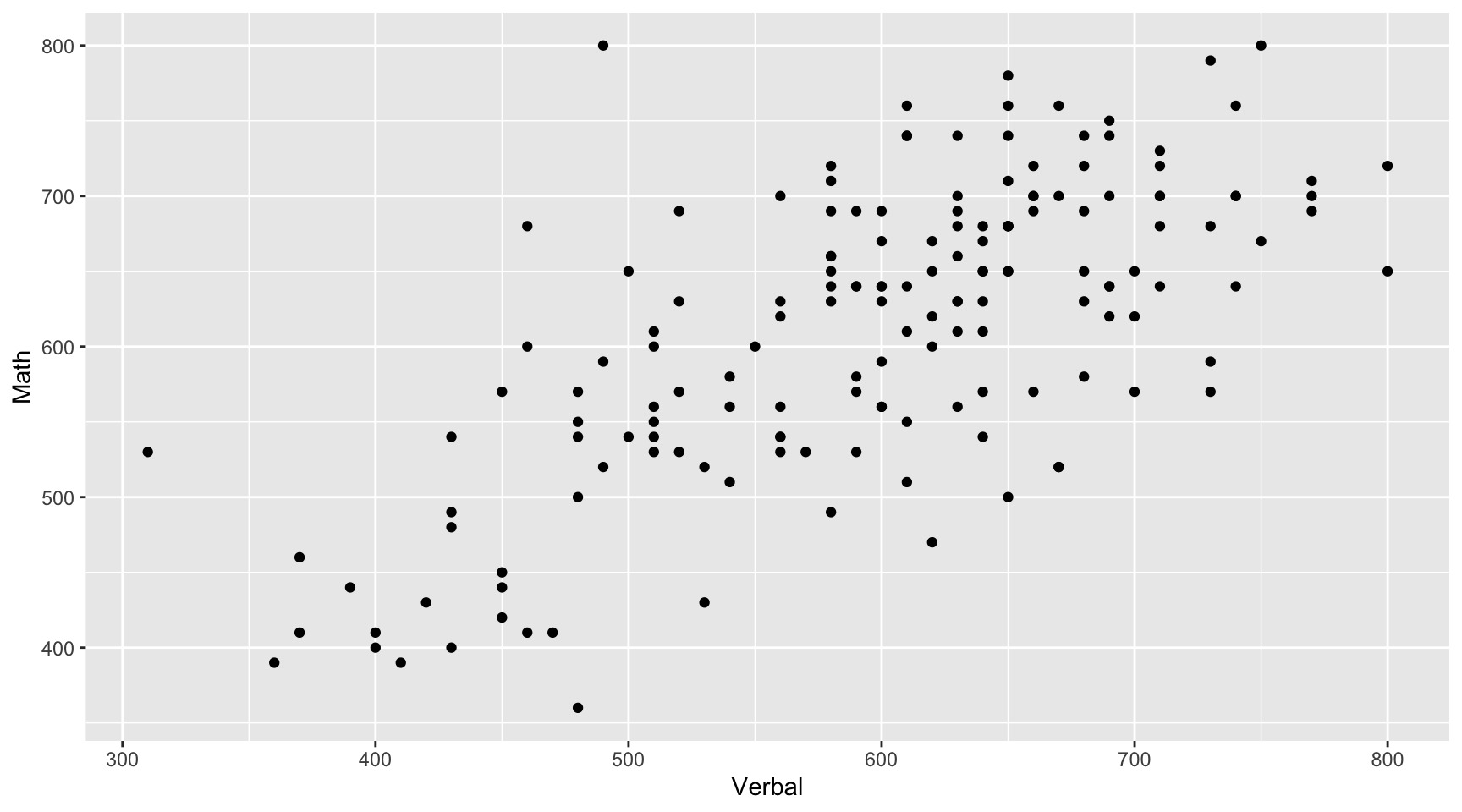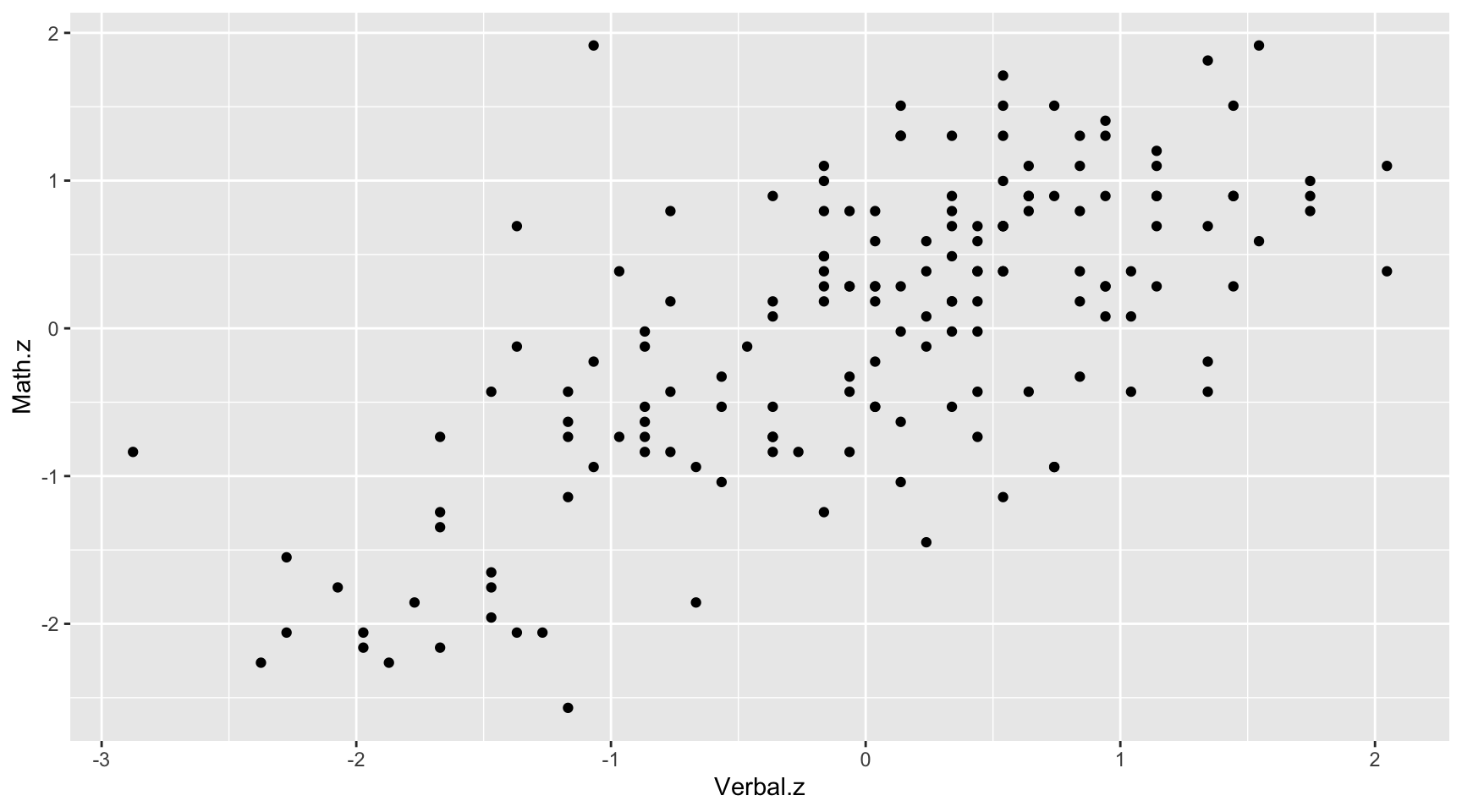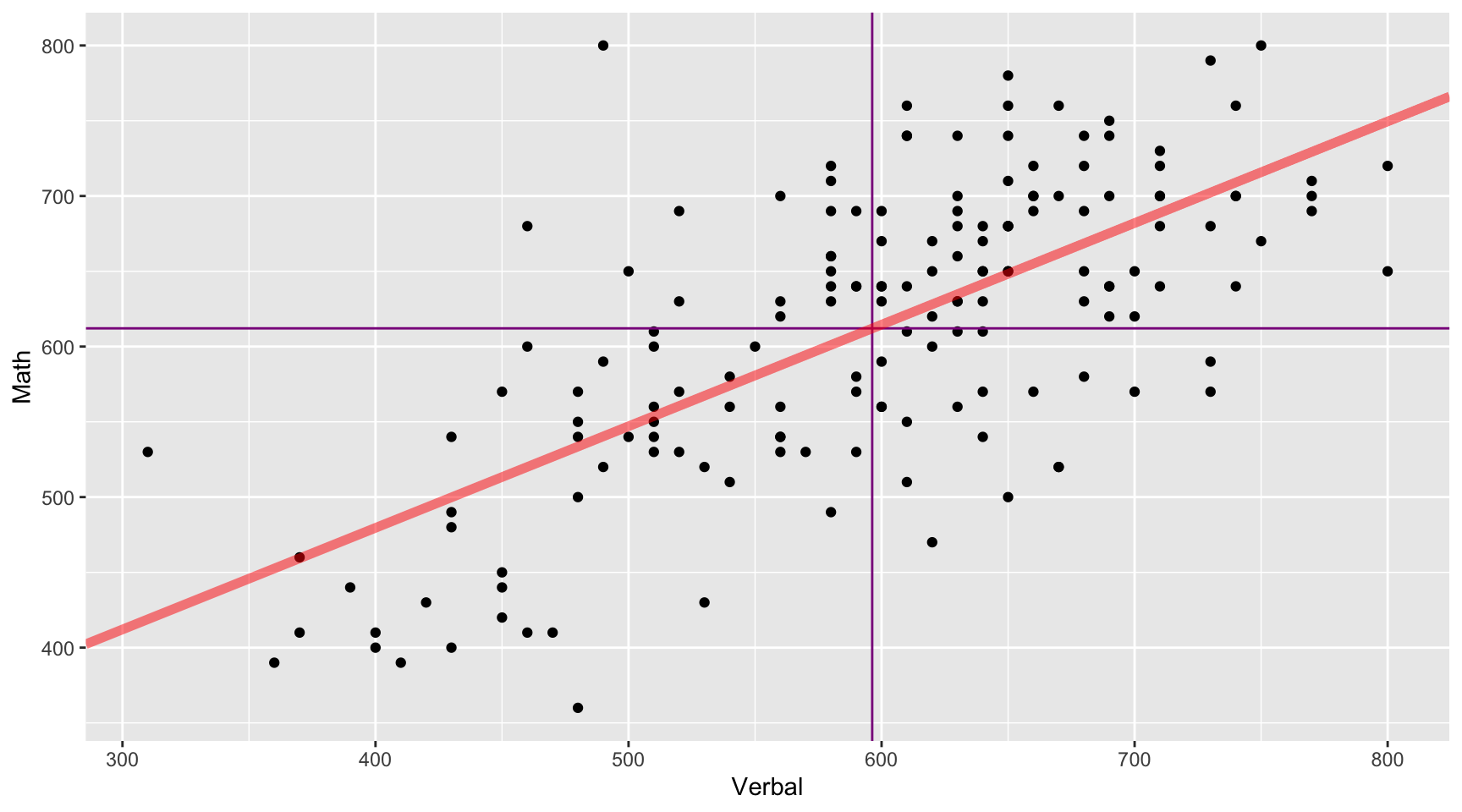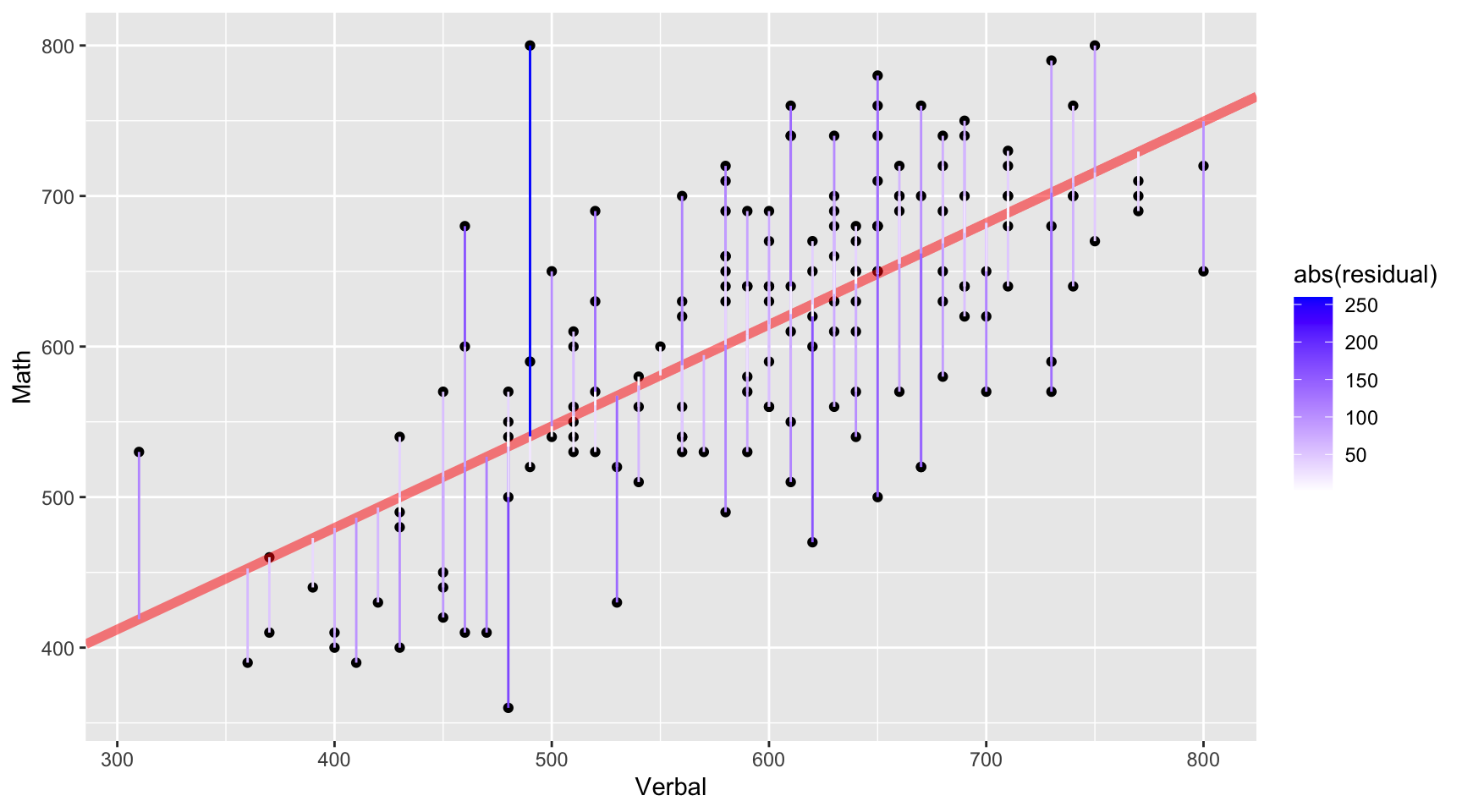March 25, 2020

## Presentations

• 7.7 Michael Munguia
• 7.13 Dennis Pong

## SAT Scores

We will use the SAT data for 162 students which includes their verbal and math scores. We will model math from verbal. Recall that the linear model can be expressed as:

$y = mx + b$

Or alternatively as:

$y = {b}_{1}x + {b}_{0}$

Where m (or $$b_1$$) is the slope and b (or $$b_0$$) is the intercept. Therefore, we wish to model:

$SAT_{math} = {b}_{1}SAT_{verbal} + {b}_{0}$

## Data Prep

To begin, we read in the CSV file and convert the Verbal and Math columns to integers. The data file uses . (i.e. a period) to denote missing values. The as.integer function will automatically convert those to NA (the indicator for a missing value in R). Finally, we use the complete.cases eliminate any rows with any missing values.

sat <- read.csv('../course_data/SAT_scores.csv', stringsAsFactors=FALSE)
names(sat) <- c('Verbal','Math','Sex')
sat$Verbal <- as.integer(sat$Verbal)
sat$Math <- as.integer(sat$Math)
sat <- sat[complete.cases(sat),]

## Scatter Plot

The first step is to draw a scatter plot. We see that the relationship appears to be fairly linear.## Descriptive Statistics

Next, we will calculate the means and standard deviations.

( verbalMean <- mean(sat$Verbal) ) ##  596.2963 ( mathMean <- mean(sat$Math) )
##  612.0988

( verbalSD <- sd(sat$Verbal) ) ##  99.5199 ( mathSD <- sd(sat$Math) )
##  98.13435
( n <- nrow(sat) )
##  162

## Correlation

The population correlation, rho, is defined as $${ \rho }_{ xy }=\frac { { \sigma }_{ xy } }{ { \sigma }_{ x }{ \sigma }_{ y } }$$ where the numerator is the covariance of x and y and the denominator is the product of the two standard deviations.

The sample correlation is calculated as $${ r }_{ xy }=\frac { { Cov }_{ xy } }{ { s }_{ x }{ s }_{ y } }$$

The covariates is calculated as $${ Cov }_{ xy }=\frac { \sum _{ i=1 }^{ n }{ \left( { X }_{ i }-\overline { X } \right) \left( { Y }_{ i }-\overline { Y } \right) } }{ n-1 }$$

(cov.xy <- sum( (sat$Verbal - verbalMean) * (sat$Math - mathMean) ) / (n - 1))
##  6686.082
cov(sat$Verbal, sat$Math)
##  6686.082

## Correlation (cont.)

${ r }_{ xy }=\frac { \frac { \sum _{ i=1 }^{ n }{ \left( { X }_{ i }-\overline { X } \right) \left( { Y }_{ i }-\overline { Y } \right) } }{ n-1 } }{ { s }_{ x }{ s }_{ y } }$

cov.xy / (verbalSD * mathSD)
##  0.6846061
cor(sat$Verbal, sat$Math)
##  0.6846061

http://bcdudek.net/rectangles

## z-Scores

Calcualte z-scores (standard scores) for the verbal and math scores.

$z=\frac { y-\overline { y } }{ s }$

sat$Verbal.z <- (sat$Verbal - verbalMean) / verbalSD
sat$Math.z <- (sat$Math - mathMean) / mathSD
head(sat)
##   Verbal Math Sex    Verbal.z      Math.z
## 1    450  450   F -1.47002058 -1.65180456
## 2    640  540   F  0.43914539 -0.73469449
## 3    590  570   M -0.06326671 -0.42899113
## 4    400  400   M -1.97243268 -2.16131016
## 5    600  590   M  0.03721571 -0.22518889
## 6    610  610   M  0.13769813 -0.02138665

## Scatter Plot of z-Scores

Scatter plot of z-scores. Note that the pattern is the same but the scales on the x- and y-axes are different.## Correlation

Calculate the correlation manually using the z-score formula:

$r=\frac { \sum { { z }_{ x }{ z }_{ y } } }{ n-1 }$

r <- sum( sat$Verbal.z * sat$Math.z ) / ( n - 1 )
r
##  0.6846061

Or the cor function in R is probably simplier.

cor(sat$Verbal, sat$Math)
##  0.6846061

And to show that the units don’t matter, calculate the correlation with the z-scores.

cor(sat$Verbal.z, sat$Math.z)
##  0.6846061

## Calculate the slope.

$m = r\frac{S_y}{S_x} = r\frac{S_{math}}{S_{verbal}}$

m <- r * (mathSD / verbalSD)
m
##  0.6750748

## Calculate the intercept

Recall that the point where the mean of x and mean of y intersect will be on the line of best fit). Therefore,

$b = \overline{y} - m \overline{x} = \overline{SAT_{math}} - m \overline{SAT_{verbal}}$

b <- mathMean - m * verbalMean
b
##  209.5542

## Scatter Plot with Regression Line

We can now add the regression line to the scatter plot. The vertical and horizontal lines represent the mean Verbal and Math SAT scores, respectively.## Examine the Residuals

To examine the residuals, we first need to calculate the predicted values of y (Math scores in this example).

sat$Math.predicted <- m * sat$Verbal + b
head(sat, n=4)
##   Verbal Math Sex    Verbal.z     Math.z Math.predicted
## 1    450  450   F -1.47002058 -1.6518046       513.3378
## 2    640  540   F  0.43914539 -0.7346945       641.6020
## 3    590  570   M -0.06326671 -0.4289911       607.8483
## 4    400  400   M -1.97243268 -2.1613102       479.5841

The residuals are simply the difference between the observed and predicted values.

sat$residual <- sat$Math - sat$Math.predicted head(sat, n=4) ## Verbal Math Sex Verbal.z Math.z Math.predicted residual ## 1 450 450 F -1.47002058 -1.6518046 513.3378 -63.33782 ## 2 640 540 F 0.43914539 -0.7346945 641.6020 -101.60203 ## 3 590 570 M -0.06326671 -0.4289911 607.8483 -37.84829 ## 4 400 400 M -1.97243268 -2.1613102 479.5841 -79.58408 ## Scatter Plot with Residuals Plot our regression line with lines representing the residuals. The line of best fit minimizes the residuals.## What does it mean to minimize the sum of squared residuals? To show that $$m = r \frac{S_y}{S_x}$$ minimizes the sum of squared residuals, this loop will calculate the sum of squared residuals for varying values of r above and below the calculated value. results <- data.frame(r=seq(r - .2, r + .2, by=.01), m=as.numeric(NA), b=as.numeric(NA), sumsquares=as.numeric(NA)) for(i in 1:nrow(results)) { results[i,]$m <- results[i,]$r * (mathSD / verbalSD) results[i,]$b <-  mathMean - results[i,]$m * verbalMean predicted <- results[i,]$m * sat$Verbal + results[i,]$b
residual <- sat$Math - predicted sumsquares <- sum(residual^2) results[i,]$sumsquares <- sum(residual^2)
}

Plot the sum of squared residuals for different slopes (i.e. r’s). The vertical line corresponds to the r (slope) calcluated above and the horizontal line corresponds the sum of squared residuals for that r. This should have the smallest sum of squared residuals.